# Methods and formulas for probability plot in Individual Distribution Identification

## Probability plot

The probability plots include:

• Points, which are the estimated percentiles for corresponding probabilities of an ordered data set.
• Middle lines, which are the expected percentile from the distribution based on maximum likelihood parameter estimates. If the distribution is a good fit for the data, the points fall along the middle line.

## Estimated probabilities

Minitab estimates the probability (P) that is used to calculate the plot points using the following methods.

• Median rank (Benard's method)
• Mean Rank (Herd-Johnson estimate)
• Modified Kaplan-Meier (Hazen)
• Kaplan-Meier product limit estimate

### Notation

TermDescription
nNumber of observations
iRank of the ith ordered observation x(i), where x(1), x(2),...x(n) are the order statistics, or the data ordered from smallest to largest

## Plot points

The middle line of the probability plot is constructed using the x and y coordinate calculations in this table.

Distribution x coordinate y coordinate
Smallest extreme value x ln(–ln(1 – p))
Largest extreme value x ln(–ln p)
Weibull ln(x) ln(–ln(1 – p))
3-parameter Weibull ln(x – threshold) ln(–ln(1 – p))
Exponential ln(x) ln(–ln(1 – p))
2-parameter exponential ln(x – threshold) ln(–ln(1 – p))
Normal x Φ–1norm
Lognormal ln(x) Φ–1norm
3-parameter lognormal ln(x – threshold) Φ–1norm
Logistic x
Loglogistic ln(x)
3-parameter loglogistic ln(x – threshold)
Gamma x Φ–1gamma
3-parameter gamma ln(x – threshold) Φ–1gamma
###### Note

Because the plot points do not depend on any distribution, they are the same (before being transformed) for any probability plot. However, the fitted line differs depending on the parametric distribution chosen.

### Notation

TermDescription
pThe estimated probability
Φ-1normValue returned for p by the inverse CDF for the standard normal distribution
Φ-1gammaValue returned for p by the inverse CDF for the incomplete gamma distribution
ln(x)The natural log of x

## Percentiles and std error of percentiles

Percentile is a value on a scale of 100 that indicates the percent of a distribution that is equal to or below that value. By default, Minitab displays tables of percentiles for parametric distribution analysis for common percentiles.

The standard errors for the percentile estimates are the square root of the variances.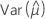,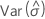,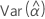,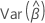,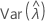,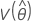,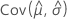,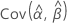, and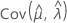denote the variances and covariances of the MLEs of μ, σ, α, β, λ, and θ taken from the appropriate element of the inverse of the Fisher information matrix.

The formulas used for percentile and variance estimates are as follows:

### Smallest extreme value distribution

Percentile
Variance

where zp = ln[–ln(1 – p)], the inverse CDF of the smallest extreme value distribution

### Largest extreme value distribution

Percentile
Variance

where zp = ln[–-ln(p)], the inverse CDF of the largest extreme value distribution

### Weibull distribution

Percentile
Variance

where zp = ln[–ln(1 – p)], the inverse CDF of the smallest extreme value distribution

### 3-parameter Weibull distribution

Percentile
Variance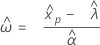where zp = ln[–ln(1 – p)], the inverse CDF of the smallest extreme value distribution

Percentile
Variance

Percentile
Variance

### Normal distribution

Percentile
Variance

where zp = the inverse CDF of the normal distribution

### Lognormal distribution

Percentile
Variance

where zp = the inverse CDF of the normal distribution

### 3-parameter lognormal distribution

Percentile
Variance

where zp = the inverse CDF of the normal distribution

### Logistic distribution

Percentile
Variance

where zp = ln[p/(1 – p)], the inverse CDF of the logistic distribution

### Loglogistic distribution

Percentile
Variance

where zp = ln[p/(1 – p)], the inverse CDF of the logistic distribution

### 3-parameter loglogistic distribution

Percentile
Variance

where zp = ln[p/(1 – p)], the inverse CDF of the logistic distribution

### Gamma distribution

Percentile
Variance

where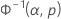is the inverse of the regularized incomplete gamma distribution

### 3-parameter gamma distribution

Percentile
Variance

whereis the inverse of the regularized incomplete gamma distribution

## Confidence limits for percentiles

Distribution Confidence limits
Smallest extreme value
Largest extreme value
Normal
Logistic
Weibull
Exponential
Lognormal
Loglogistic
3-parameter Weibull
If λ < 0:
If λ ≥ 0:
2-parameter exponential
If λ < 0:
If λ ≥ 0:
3-parameter lognormal
If λ < 0:
If λ ≥ 0:
3-parameter loglogistic
If λ < 0:
If λ ≥ 0:

### Notation

TermDescription
KγThe (1 + γ) / 2 percentile of a standard normal distribution
By using this site you agree to the use of cookies for analytics and personalized content.  Read our policy